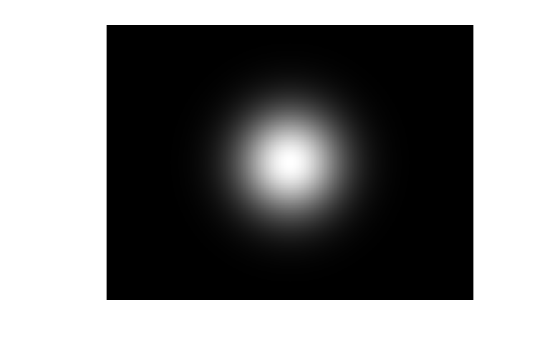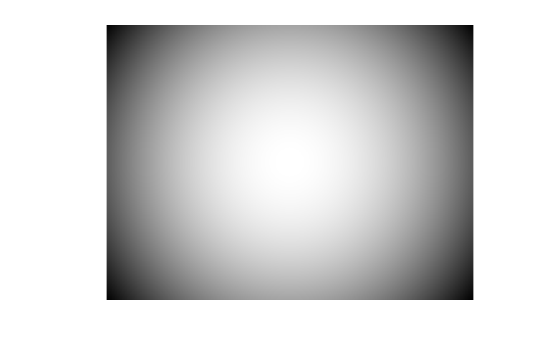# Increase Filter Strength Radially Outward

This example shows how to create and apply filters whose strength increases radially outward.

```I = imread('peppers.png'); I = im2double(I); imshow(I)```### Blur Image Using Gaussian Weighting Function

Create a blurry copy of the image using a Gaussian filter with standard deviation of 2.

```Iblurred = imgaussfilt(I,2); imshow(Iblurred)```Create a weight image as a Gaussian filter of the same size of the image. To increase the portion of the image that appears sharp, increase the value of `filterStrength`.

```filterStrength = 50; weights = fspecial('gaussian',[size(I,1) size(I,2)],filterStrength); imshow(weights,[])```Normalize the weight image to the range [0, 1] by using the `rescale` function.

`weights = rescale(weights);`

Create a weighted blurred image that is a weighted sum of the original image and blurry image. MATLAB automatically replicates the weight matrix for each of the R, G, and B color channels.

`IweightedBlurred = I.*weights + Iblurred.*(1-weights);`

Display the result. The image is sharp in the center and becomes more blurry radially outwards. To increase the portion of the image that appears sharp, increase the value of

`imshow(IweightedBlurred)`### Vignette Image Using 1/R^2 Weighting Function

Get the size of the image.

```sizex = size(I,2); sizey = size(I,1);```

Specify the center of the vignette.

```xcenter = size(I,2)/2; ycenter = size(I,1)/2;```

Define the x- and y-coordinates of the surface.

`[X,Y] = meshgrid((1:sizex)-xcenter,(1:sizey)-ycenter);`

Define the radius from the center at each (x,y) coordinate.

`R2 = X.^2 + Y.^2;`

Define the weighting function as the inverse of R, scaled to the range [0, 1].

```R2 = rescale(R2); weights = (1-R2); imshow(weights)```Apply the weighting function to the image and display the result.

```I2 = I.*weights; imshow(I2)```